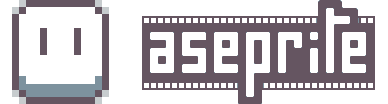# Centralization tool

Can we get a centralization tool? Something that shows where the center of the canvas is and also allow us to move a selection to the cente? I spend so much time drawing lines and couting pixels to find the center and i think that a tool like that would be really cool, useful and time saving!

2 Likes

I’ve only been using asesprite since earlier today, and I also think this would be useful. I put together a quick script that does it.

You can grab my script from this git repository.

Here is the current content for anyone who just wants to look… if you want to use it, go to the repository, as that is where I will put any improvements I make:

``````----------------------------------------------------------------------
-- Center the current selection on the sprite
-- Copyright 2020 Geoff Beier <geoff@tuxpup.com>
----------------------------------------------------------------------

-- bail if there's no active sprite
local spr = app.activeSprite
if not spr then return end

-- bail if nothing's selected
local sel = spr.selection
if sel.isEmpty then return end

--print("sel:"..sel.bounds.x..","..sel.bounds.y)

-- The MoveMask command requires us to specify X <units> up, Y <units> down
-- rather than just specifying new coordinates.
new_x = spr.bounds.width/2 - sel.bounds.width/2
x_dir = "right"
x_distance = 0
if new_x < sel.bounds.x then
x_dir = "left"
x_distance = sel.bounds.x - new_x
elseif new_x > sel.bounds.x then
x_dir = "right"
x_distance = new_x - sel.bounds.x
end

new_y = spr.bounds.height/2 - sel.bounds.height/2
y_dir = "down"
y_distance = 0
if new_y < sel.bounds.y then
y_dir = "up"
y_distance = sel.bounds.y - new_y
elseif new_y > sel.bounds.y then
y_dir = "down"
y_distance = new_y - sel.bounds.y
end

--print('need to move ' .. x_dir .. ' ' .. tostring(x_distance) .. ' and ' .. y_dir .. ' ' .. tostring(y_distance))

if x_distance ~= 0 then
direction=x_dir,
units="pixel",
quantity=x_distance
}
end

if y_distance ~= 0 then